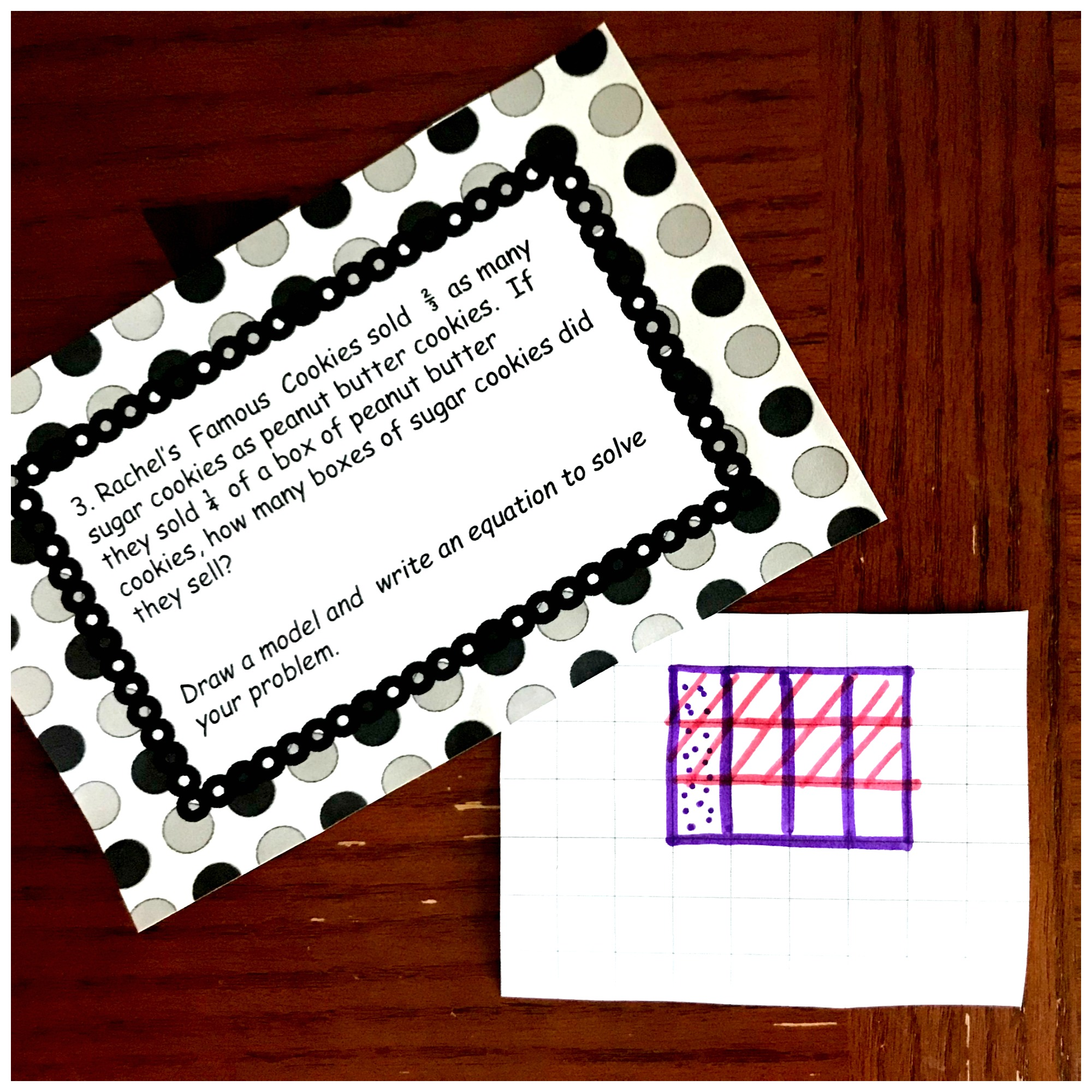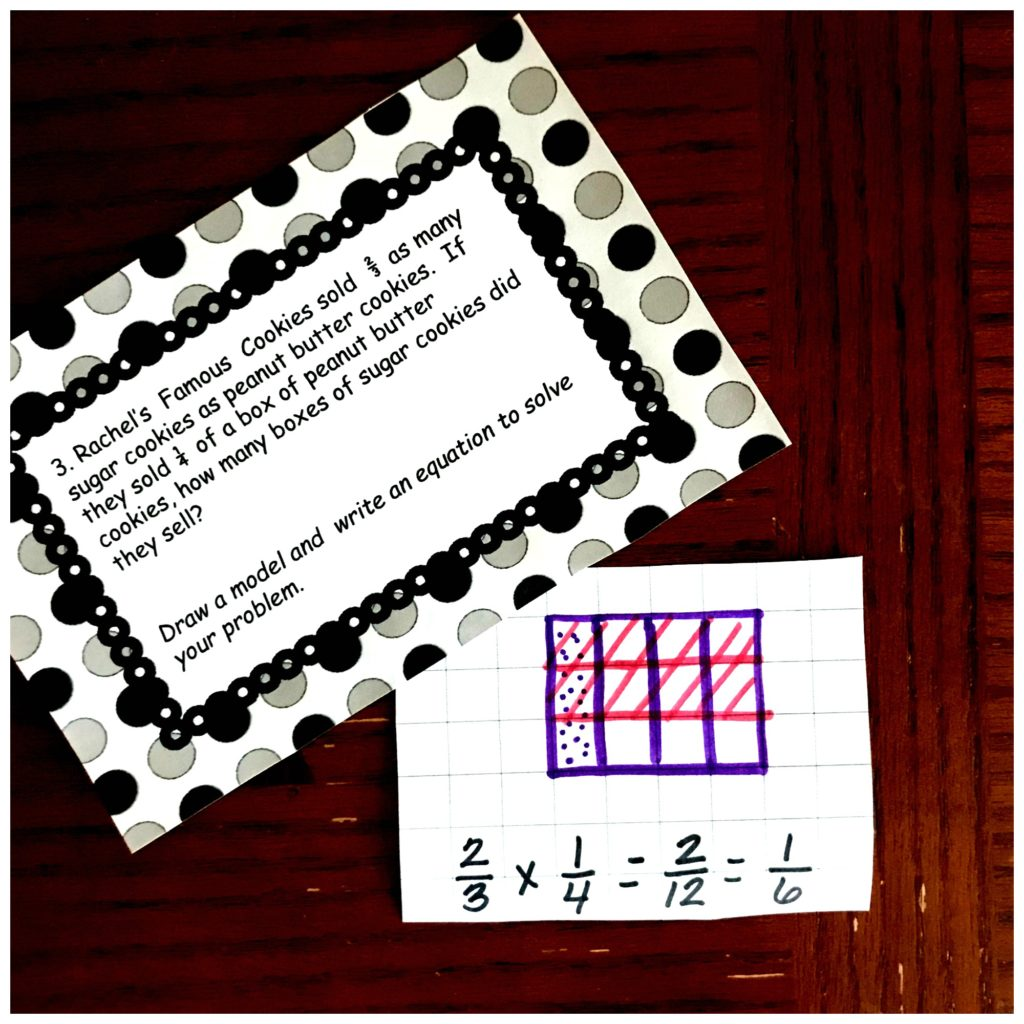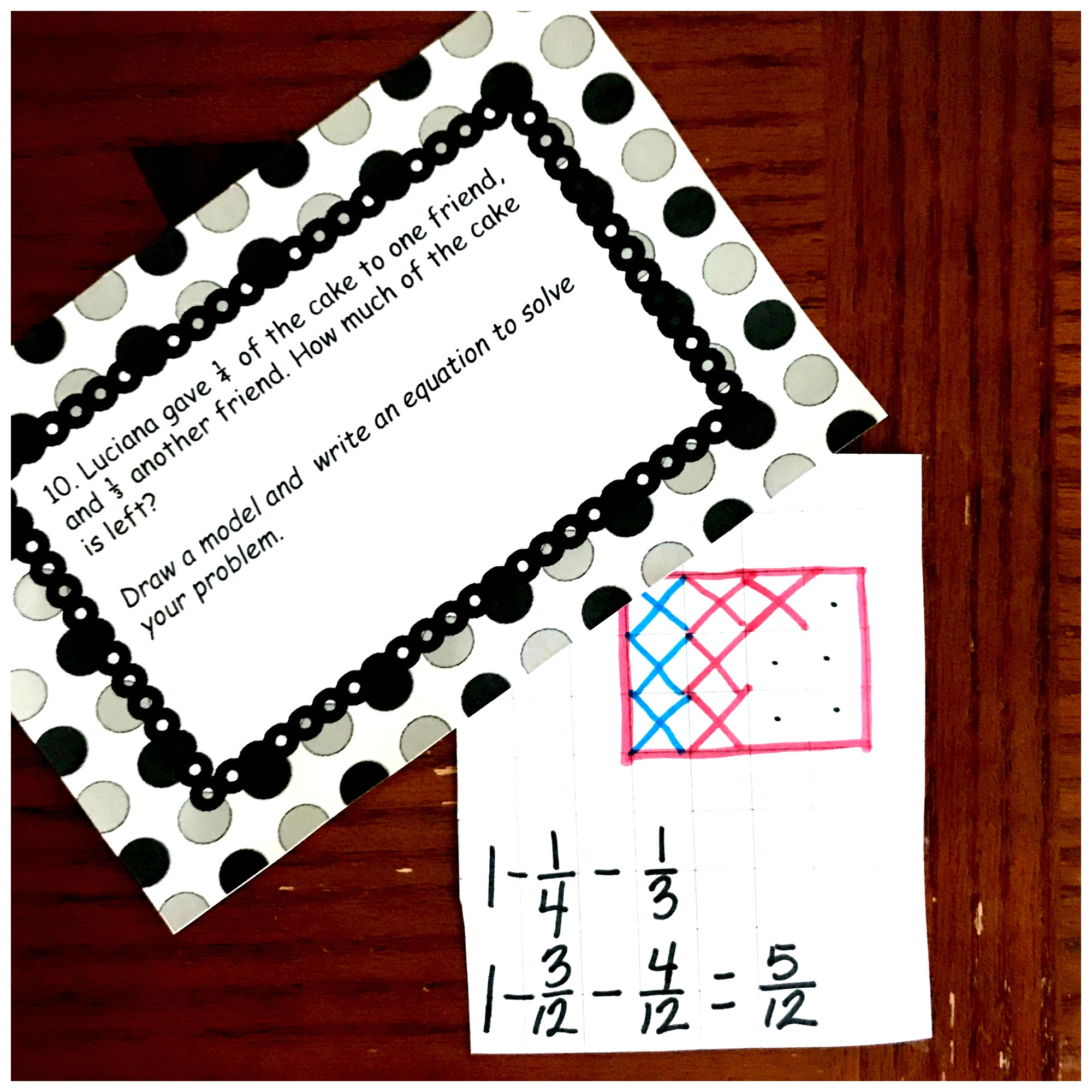Home » Math » 10 Multiplying Fractions Word Problems For Some Real Life Examples

# 10 Multiplying Fractions Word Problems For Some Real Life Examples

These 10 multiplying fractions word problems are a great way to work on multiplying fractions using real-life examples. Get them FREE today.Have you ever heard, “I will never use this”?

It is important for children to see how math is used in everyday life. And word problems are one way to do this.

Multiplying Fractions seems like a foreign concept to many students, but it is used.

Have you ever wanted to only make 1/2 of a recipe? If so, you have probably multiplying fractions…..especially if the recipe called for 3/4 a cup of flour.

So today, it is time to finish up our multiplying fraction unit with some word problems.

Grab our other free multiplying fractions by fractions activities.

How to Multiply Fractions by Fractions – Step by Step Instructions with Free Printable

Here is a Multiplying Fractions BINGO Game That’s Perfect for Extra Practice

3 Cut and Paste Worksheets For Multiplying Fractions Practice

Free Printable Fraction Game For Multiplying Fractions

Or download them all in one easy step.

## Prep – Work

These task cards are easy to prepare.

1. Print off on copy paper.
2. Have children cut them out and glue them in their math journal.
3. Finally, provide them pencils, glue, and colored pencils. and you are ready to go.

## Multiplying Fractions Word Problems Sample

Rachel’s  Famous  Cookies sold  2/3 as many sugar cookies as peanut butter cookies.  If they sold 1/4  of a box of peanut butter cookies, how many boxes of sugar cookies did they sell?

The first step is to look at we know. We know that they sold 1/4 a box of peanut butter cookies. But they only sold 2/3 of the 1/4 when it came to the sugar cookies.

So if we want to start with a diagram, we can begin by drawing a box of cookies and coloring in 1/4.Next, we can color in 2/3.Finally, we look and see what part overlaps, and this is the answer!  Two out of the 12 squares overlap, so our answer is 2/12 or 1/6 when it is simplified.## Changing Things Up a Bit

I’m a firm believer that children should not be given word problems that require the same operation. That is not real life, and that does teach them to learn what needs to be done to solve the problem. So even though this printable is for practicing multiplying fractions with word problems there are a few word problems where multiplication is not used. Here is one example.

Luciana gave 1/4 of the cake to one friend, and 1/3 another friend. How much of the cake is left if she started with a whole cake?

This word problem has the children subtracting to figure out the answer.

1. We know that Luciana started with a whole cake, which equals one. So we want to begin by drawing a square.

2. The next thing we have to figure out is how many parts we need to divide it up into. Since we now we will be subtracting 1/4 and 1/3 we will need to get the Least Common Multiple. The multiples of three and four are…..

4: 4, 8, 12

3: 3, 6, 9, 12

As you can see 12 is the least common multiple. So our square needs to have 12 equal parts.

3. Next, we will need to find equivalent fractions for both of our fractions.

1/4 x 3/3 = 3/12 and 1/3 x 4/4 = 4/12.

4. Now all that is left is to subtract 3 part and then 4 parts.

5. Finally, we can see that there 5/12 of the cake left.Word Problems are an important part of math instruction, and how we do math in everyday life. Enjoy working through these problems with your children.

You’ve Got This

Rachel

You may also like: